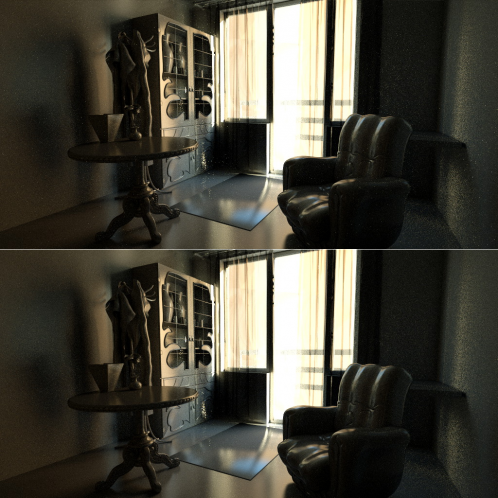# ”Defly” – программа удаления горячих пикселов/”святлечков”Before/After (scaled)
###### Компьютерная программа, 2010

`Извините, данная страница доступна только на английском языке.`

Update: please note that now Blender supports internal denoisers that are way more sophisticated and normally work better! This page is kept for archival purposes.

Defly is an image filtering tool for removing fireflies (hot pixels) from Cycles, Octane and other computational renderings. Although this tool may help you, beware that it is not intended as an everyday production tool, but rather a proof of concept experiment.

Even though Octane has a built-in noise reduction (that seems to be based on principle explained here) it’s sometimes useful to be able to do filtering independently from rendering, to avoid lost details during animation or re-rendering animation, so I decided to keep this online for now.

Please report issues in comments below. Thank you.

Warning: Read agreement first! This program is provided as is with no any sort of warranties, so don’t put your life on it.
As there’ve been not much users noticed program is for Windows only.

How to use:
Important note: jpg causes a little blur which reduces effectiveness!
Image should be rendered without any filtering for same reason!
This was not tested, but this tool should be useless for digital camera firefly removal.

1. Load image or sequence frame that has most fireflies and sharp details
and navigate (in lower viewport) with arrow buttoons to a place that suffers from fireflies most.

2. Adjust “Remove firefly” slider so that you remove all firefly and maybe a little of brighter noise as needed:

3. Adjust “Preserve detail” slider so that you gain back thin lines or other small details that were removed by previous step if you had any:
(do not overdo with “Remove firefly” as it will require more of “Preserve details” parameter which in turn will reduce effectiveness of first one)

4. If you are doing single image press “Save this image”… Entering file name don’t forget to set extension as it will define file format.
If you are doing sequence do not save anything. Do not change parameters.
Open sequence frame that you want removal (sequence start and end filenames should appear beneath buttons)
to start with and press “Process frame range”. Frame number and extension will be added automaticaly (*.png)

Hot pixel removal principle explained:
– Program processes all non-boundary pixels of image one by one on r,g,b channels as averaged value or separated.
– If pixel is more than inverted “Remove…” value brighter than surrounding (left, right, top, bottom) pixels it is replaced with their average value if following condition is met:
– The difference between brightest and darkest pixels around (8 neighbouring pixels) must be smaller than “Preserve…” value.

Agreement:
By downloading, storing or using this program you are entering the following agreement:

You use this program at your own risk! This program comes with no warranty whatsoever.
Author is not liable for any direct/indirect damages caused by operation or inoperation or quality of this program.
You may not sell or distribute this program for any reward.
You may not separate the program from “Readme.txt” file and/or remove agreement.

Defly v0.9
Copyright © 2010 Konstantins Visnevskis, All rights reserved.

Agree and Download (~1MB)

Source code:
Note: the program was intended as a quick temporary fix.

[+] Показать

[-] Спрятать

``````unsigned char p;//channel,x,y
int mx, my, sli, sl2, cop, a, act;
mx = img.GetWidth();
my = img.GetHeight();
sli = 255-Slider1->GetValue();
//Firefly threshold
sl2 = 255-Slider2->GetValue();
//Detail preserve threshold
unsigned char *gpic=(unsigned char*)malloc(mx*my*3*sizeof(unsigned char));
gpic=img.GetData();
unsigned char *spic=(unsigned char*)malloc(mx*my*3*sizeof(unsigned char));
memcpy(spic,gpic,mx*my*3);

if(!CheckBox1->GetValue())cop=0;else cop=1;
//evaluate RGB channels separately? (colorful fireflies?)

for(int x=1;x<mx-2;x++)
{
for(int y=1;y<my-2;y++)
{   //load pixels
lo=255;lo=255;lo=255;
hi=0;hi=0;hi=0;

p=gpic[3*((y-1)*mx+(x))];
check(p,0);
p=gpic[3*((y)*mx+(x-1))];
check(p,0);
p=gpic[3*((y)*mx+(x))];
p=gpic[3*((y)*mx+(x+1))];
check(p,0);
p=gpic[3*((y+1)*mx+(x))];
check(p,0);

p=gpic[3*((y-1)*mx+(x-1))];
check(p,0);
p=gpic[3*((y+1)*mx+(x-1))];
check(p,0);
p=gpic[3*((y-1)*mx+(x+1))];
check(p,0);
p=gpic[3*((y+1)*mx+(x+1))];
check(p,0);

p=gpic[3*((y-1)*mx+(x))+1];
check(p,1);
p=gpic[3*((y)*mx+(x-1))+1];
check(p,1);
p=gpic[3*((y)*mx+(x))+1];
p=gpic[3*((y)*mx+(x+1))+1];
check(p,1);
p=gpic[3*((y+1)*mx+(x))+1];
check(p,1);

p=gpic[3*((y-1)*mx+(x-1))+1];
check(p,1);
p=gpic[3*((y+1)*mx+(x-1))+1];
check(p,1);
p=gpic[3*((y-1)*mx+(x+1))+1];
check(p,1);
p=gpic[3*((y+1)*mx+(x+1))+1];
check(p,1);

p=gpic[3*((y-1)*mx+(x))+2];
check(p,2);
p=gpic[3*((y)*mx+(x-1))+2];
check(p,2);
p=gpic[3*((y)*mx+(x))+2];
p=gpic[3*((y)*mx+(x+1))+2];
check(p,2);
p=gpic[3*((y+1)*mx+(x))+2];
check(p,2);

p=gpic[3*((y-1)*mx+(x-1))+2];
check(p,2);
p=gpic[3*((y+1)*mx+(x-1))+2];[collapse]
check(p,2);
p=gpic[3*((y-1)*mx+(x+1))+2];
check(p,2);
p=gpic[3*((y+1)*mx+(x+1))+2];
check(p,2);

//calc average around
a=(int)((int)p+(int)p+(int)p+(int)p)/4;
a=(int)((int)p+(int)p+(int)p+(int)p)/4;
a=(int)((int)p+(int)p+(int)p+(int)p)/4;

act=0;
if(cop==1)
{
if(((int)p-(int)a>sli) && (hi-lo<sl2))
{p=(unsigned char)a;act++;}
if(((int)p-(int)a>sli) && (hi-lo<sl2))
{p=(unsigned char)a;act++;}
if(((int)p-(int)a>sli) && (hi-lo<sl2))
{p=(unsigned char)a;act++;}
}
else
{
if(((int)p-(int)a>sli ||
(int)p-(int)a>sli ||
(int)p-(int)a>sli) && (hi+hi+hi-lo-lo-lo<sl2*3))
{p=(unsigned char)a;act++;
p=(unsigned char)a;act++;
p=(unsigned char)a;act++;}
}

if(act>0)
{
spic[3*((y)*mx+(x))]=p;
spic[3*((y)*mx+(x))+1]=p;
spic[3*((y)*mx+(x))+2]=p;
}
}
}
img.SetData(spic);
``````

Комментарии (13):

Ayax says:

Отличная утилита. Экономит кучу времени.

Volodymyr M. says:

This tool is great at firefly removal! Thanks for taking the time to make this.

kwon hyuk jin says:

thanks

Muhammet Emin Öner says:

Hello friend!
Very useful utility, thanks!

DigitalTouch says:

You sir, made my day.
Thank you for the tools.

tester says:

hello, is there a mac version?

@tester Hi, unfortunately no. I made it long ago and there was no big demand so I left it as is. Not sure I even can compile it anymore.
Anyhow, I’m wondering, why something like this still is not implemented in Blender. The principle is really simple.

jit says:

thanks

Max Lesnik says:

Спасибо огромное! Это офигенно! Сэкономил кучу часов рендера!

paolo says:

compliments! it improves a lot my renderings!
thanks

This sounds like a very useful tool! Saw your link from BlenderNation today. I’ll check it out! Thank you!

Leonardo Chaparro says:

Thanks

Konstantins Visnevskis says:

You’re welcome.